• java 二维数组矩阵乘法的实现方法，需要的朋友可以参考一下
• //二维数组存储矩阵乘法 void multi(int a, int b){ for (int i=0;i;i++) //i代表a矩阵的行数 { for (int j=0;j;j++) //j代表b矩阵的列数 { for (int k=
#include <iostream>
using namespace std;
int c={0};
int d={0};
//二维数组存储矩阵乘法
void multi(int a, int b){
for (int i=0;i<3;i++)			//i代表a矩阵的行数
{
for (int j=0;j<2;j++)		//j代表b矩阵的列数
{
for (int k=0;k<3;k++)	//k代表a矩阵的列数和b数组的行数
{
c[i][j]+=a[i][k]*b[k][j];
}
}
}
}
//一维数组存储矩阵乘法
void multi2(int a, int b){
for (int i=0;i<3;i++)			//i代表a矩阵的行数
{
for (int j=0;j<2;j++)		//j代表b矩阵的列数
{
for (int k=0;k<3;k++)	//k代表a矩阵的列数和b数组的行数
{
//一个矩阵用一维数组存储时(假设该矩阵为m*n的)，则
//a[i][j]在这个一维数组中的位置为array[n*i+j]
d[2*i+j]+=a[3*i+k]*b[2*k+j];
}
}
}
}

int main(){
//使用一维数组进行矩阵乘法运算
int a1={1,1,1,2,2,2,3,3,3};
int b1={1,2,3,4,5,6};
//for (int i=0;i<6;i++)
//	d[i]=0;
multi2(a1,b1);
for (int i=0;i<6;i++)
cout << d[i] << " ";
cout << endl;

//使用二维数组进行矩阵乘法运算
int a2={{1,1,1,},{2,2,2},{3,3,3}};
int b2={{1,2},{3,4},{5,6}};
//for (int i=0;i<3;i++)
//{
//	for (int j=0;j<2;j++)
//		c[i][j]=0;
//}
multi(a2,b2);
for (int i=0;i<3;i++)
{
for (int j=0;j<2;j++)
cout << c[i][j] <<" ";
cout << endl;
}
return 0;
}

展开全文c
• php 笛卡尔积二维数组矩阵算法 生成多个组合 php 笛卡尔积二维数组矩阵算法 生成多个组合 php 笛卡尔积二维数组矩阵算法 生成多个组合 php 笛卡尔积二维数组矩阵算法 生成多个组合 php 笛卡尔积二维数组矩阵...
• 知识回顾 若A为NxM阶矩阵 B为MxP阶矩阵，二者相乘之后的矩阵则为...设进行矩阵乘法的两个矩阵为a 3x3型矩阵和b 3x2型矩阵 我们在运用数学思维计算矩阵乘法时首先计算的便是 z = a*b+a*b
知识回顾
若A为NxM阶矩阵 B为MxP阶矩阵，二者相乘之后的矩阵则为NXP阶矩阵 运算过程：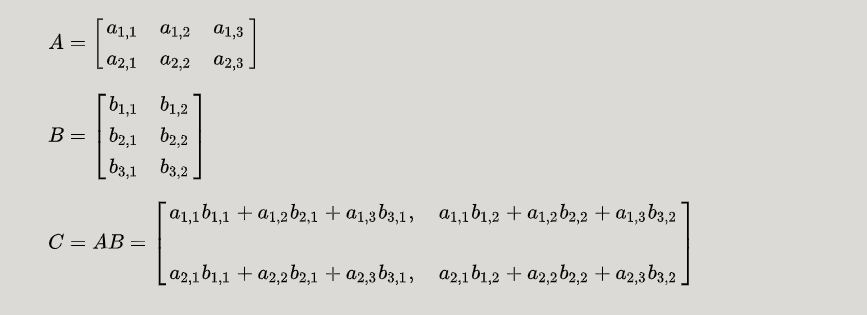思路
计算并显示出来这个结果 我们则需要 一个计算方法 和 一个显示方法因此主函数可以写的相当之简单 ， 键入想要求解的矩阵的数值 然后调用两种方法即可
显示方法
只需要循环显示出行列值然后分行即可
计算方法
设进行矩阵乘法的两个矩阵为a 3x3型矩阵和b 3x2型矩阵
我们在运用数学思维计算矩阵乘法时首先计算的便是 z = a*b+a*b+a*b 转换成代码则可想到 矩阵可以利用二维数组来实现 下标的改变以及算术累加可以用for循环来实现在此之上我们可以再思考 z计算过后我们将会继续计算z 而列下标的改变则可再次利用循环来实现 以此类推矩阵第一行的数值计算过后 我们要转为求解第二行的数值 这时则可再次利用循环来实现行的改变
因此 二维数组实现矩阵乘法的基本思路就是 利用嵌套循环来实现数值的累加计算，矩阵列以及行的变化。
以下为实现代码：
public class Example4_04{
public static void main(String[] args){
int[][] a={{-4 , 5 , -7},
{2, -3 , -4},
{3 , 4 , 5}};
int[][] b={{1 , 2},
{5 , 6},
{-7 , 9},};
int[][] result = mul(a,b);
print(result);
}
//mul返回值的类型是一个二维数组
static int[][] mul(int[][] x , int[][] y)
{
int i , j , k;//循环控制变量
//申请结果数组的空间
//行长度为x数组的行长度，列长度为y数组第一行的行长度
int[][] z=new int[x.length][y.length];
for(i = 0 ; i < x.length ; i++)
{	for(j = 0 ; j < y.length ; j++)
{
z[i][j] = 0;
for(k = 0 ; k < x.length ; k++)
z[i][j] = z[i][j] + x[i][k] * y[k][j];
}
}
return z;
}
static void print(int[][] x){
int i , j;
for(i = 0 ; i < x.length ; i++){
for(j = 0 ; j < x[i].length ; j++)
System.out.printf("%5d",x[i][j]);
System.out.println();
}
}
}


展开全文算法 java 线性代数
• 进行二维数组乘法时，如果数组长度为十万及百万以上时，如何防止溢出？
• 适合初学java程序设计的新手。分别使用一维、二维数组的方法对乘法表打印实现
• 问题一：二维数组转置问题； 代码如下： #include &amp;amp;lt;stdio.h&amp;amp;gt; #include &amp;amp;lt;stdlib.h&amp;amp;gt; /* run this program using the console pauser or add your ...
问题一：二维数组转置问题； 代码如下：
#include <stdio.h>
#include <stdlib.h>
/* run this program using the console pauser or add your own getch, system("pause") or input loop */
void shift(int (*a),int ,int );
int main(int argc, char *argv[]) {
/*矩阵的转置，实现将m行n列的矩阵实现转置（通俗点来说就是行列互换）
1，本题感觉用二维数组更为简便；
2，设置指针数组，指针数组每一个成员分别直线每一行的起始位置，然后依次累加；
*/
int i,j,m;
int n=4;
printf("注意：该转置函数仅适用于4列的二维数组，若要改变列数，可改变以上n的值，以及方法传参中行指针中数组的值；\n");
printf("请输入二维数组的行数：");
scanf("%d",&m);
printf("\n");
int a[m][n];
for(j=0;j<m;j++){
for(i=0;i<n;i++){
scanf("%d",&a[j][i]);
}
}
printf("输出转置后的数列：\n");
shift(a,m,n);
return 0;
}
void shift(int (*a),int m,int n){             //第一次出错的地方是参数中的int (*a)，我写的是int (*a),这
//是对于二维数组指针传参的不理解；这是行指针的定义格式，每行由多少个元素，后边就由多大的数组；
//可以分解成a,a,a-----a[n],方便理解；
int i,j,k;
for(i=0;i<n;i++){
for(j=0;j<m;j++){
printf("%d  ",*(a[j]+i));
}
printf("\n");
}
}
第一次出错原因：出错的地方是参数中的int (*a)，我写的是int (*a)这是对于二维数组指针传参的不理解；这是行指针的定义格式，每行有多少个元素，后边就由有大的数组；可以分解成a,a,a-----a[n],方便理解； 出现问题：如果要实现任意行列的转置，在方法的参数值无法定义列的任意值，设置输入的任意值列为静态变量也不行，这里不知道如何解决； 但是可以在不用多余函数的情况下，仅仅在main函数中写出所有的代码即可实现；方法代码在以下运行结果后； 运行结果：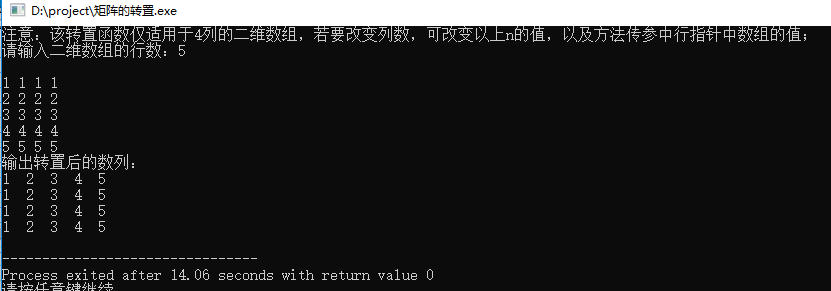方法二： 代码如下：
#include <stdio.h>
#include <stdlib.h>
/* run this program using the console pauser or add your own getch, system("pause") or input loop */
int main(int argc, char *argv[]) {
int i,j,m,n;
printf("请输入二维数组的行数：");
scanf("%d",&m);
printf("请输入二维数组的列数：");
scanf("%d",&n);
printf("\n");
int a[m][n];
printf("输入数列：\n");
for(j=0;j<m;j++){
for(i=0;i<n;i++){
scanf("%d",&a[j][i]);
}
}
int b[n][m];
int k,l;
printf("输出转置后的数列：\n");
for(k=0;k<n;k++){
for(l=0;l<m;l++){
b[k][l]=a[l][k];
printf("%d  ",b[k][l]);
}
printf("\n");
}
return 0;
}
运行结果：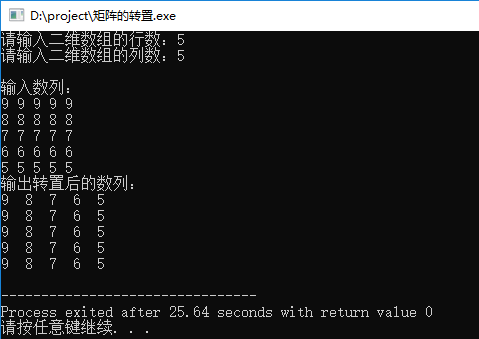问题二： 矩阵的乘法运算 矩阵的乘法运算：
依照于数学的运算法则给定两个矩阵；定义一个新的二维数组，新二维数组的行数是第一个矩阵的行数，二维数组的列数是第二个矩阵的列数；根据运算法则求值存储；
详细理解见代码中分析； 运行代码：
#include <stdio.h>
#include <stdlib.h>
/* run this program using the console pauser or add your own getch, system("pause") or input loop */
int main(int argc, char *argv[]) {
/*矩阵的乘法运算：1，依照于数学的运算法则给定两个矩阵；
2，定义一个新的二维数组，新二维数组的行数是第一个矩阵的行数，二维数组的列数是第二个矩阵的列数；
3，根据运算法则求值存储；
*/
int a1,a2,a3,a4;
printf("输入第一个矩阵的行数：");       //输入第一个矩阵
scanf("%d",&a1);
printf("输入第一个矩阵的列数：");
scanf("%d",&a2);
int a[a1][a2];
int i1,j1;
for(i1=0;i1<a1;i1++){
for(j1=0;j1<a2;j1++){
scanf("%d",&a[i1][j1]);
}
}
printf("\n");
printf("输入第二个矩阵的行数：");       //输入第二个矩阵
scanf("%d",&a3);
printf("输入第二个矩阵的列数：");
scanf("%d",&a4);
int b[a3][a4];
int i2,j2;
for(i2=0;i2<a3;i2++){
for(j2=0;j2<a4;j2++){
scanf("%d",&b[i2][j2]);
}
}
if(a2!=a3){
printf("两矩阵不满足相乘的基本条件；");
}else{
printf("输出相乘后的矩阵：\n");
int c[a1][a4];
int i,j;
int m,n;
int k,l;                                  //数组的每一位赋0
for(k=0;k<a1;k++){
for(l=0;l<a4;l++){
c[k][l]=0;
}
}
for(i=0;i<a1;i++){
for(j=0;j<a4;j++){
for(m=0;m<a2;m++){
c[i][j]+=a[i][m]*b[m][j];         //超级关键
}
printf("%d  ",c[i][j]);
}
printf("\n");
}
}
return 0;
}
切记关键代码c[i][j]+=a[i][m] * b[m][j]；见代码 运行结果：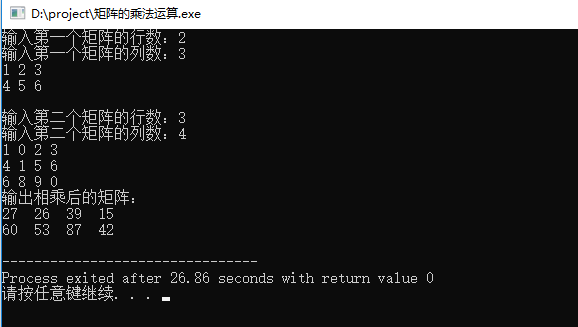展开全文• java二维数组的个数Here, We have to find the multiplication of the two matrix by moving forward direction in row major (row by row) order in one matrix and by backward direction in row major order in ...

java二维数组的个数
Here, We have to find the multiplication of the two matrix by moving forward direction in row major (row by row) order in one matrix and by backward direction in row major order in second one i.e. multiplying first element of one matrix with the last element of second one, second element of first one with the second last element of second one and so on…
在这里，我们必须通过以下方式找到两个矩阵的乘法：在一个矩阵中按行主要(行)顺序向前移动，在第二个矩阵中按行主要顺序向后移动，即将一个矩阵的第一个元素与最后一个元素相乘第二个元素的第一个元素，第二个元素的倒数第二个元素的第二个元素，依此类推…
Example:
例：
First matrix (X)
8	    9	     5
5	    6	     3
4	    9	     7

Second matrix (Y)
6	     8	   10
12	     4	    5
3	    17	   20


We have to multiply like that,
我们必须那样繁衍，
First element of first matrix (X) with the last one of second matrix (Y) → 8*20 and save it to the first index of new matrix. Then , 9*17 and save it to the 2nd index of new one and so on...
第一个矩阵( X )的第一个元素与第二个矩阵( Y )的最后一个元素→8 * 20，并将其保存到新矩阵的第一个索引中。 然后，9 * 17并将其保存到新索引的第二个索引，依此类推...
The new Matrix Z is
新的Matrix Z是
    160		153		15
24		25		36
40		72		42


Program:
程序：
public class Multiplication
{
public static void main(String[] args)
{
//first matrix
int x[][]={{8,9,5},{5,6,3},{4,9,7}};
//second matrix
int y[][]={{6,8,10},{12,4,5},{3,17,20}};
//matrix which stores multiplication of the two
int z[][]=new int;

for(int i=0,j=2;i<3;i++,j--)
for(int k=0,l=2;k<3;k++,l--)
z[i][k]=x[i][k]*y[j][l];

for(int i=0;i<3;i++)
{
for(int j=0;j<3;j++)
System.out.print(z[i][j]+" ");
System.out.println();
}
}
}


Output
输出量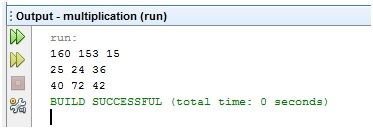翻译自: https://www.includehelp.com/java-programs/print-multiplication-of-two-2-d-arrays-by-traversing-forward-direction.aspx

java二维数组的个数

展开全文• #include <stdio.h> int main() { int a,b,c,i,j,k; printf("输入a:\n");...i++) //输入数组a for(j=0;j<3;j++) scanf("%d",&a[i][j]); printf("输入...C
• #include #include #include int main() { int **p1,**p2,**p; int a,b,c; int i,j,k; scanf("%d%d%d",&a,&b,&c);...//输入一个a*b 和 b*c的矩阵，做矩阵相乘。 p1=(int **)malloc(sizeof (int*)*a); p2=(int **)ma
• C语言 实在 矩阵乘法 加法 转置 各个子涵数都有通用性，内存的分配是使用二维数组动态 malloc 分配
• ## 二维数组矩阵相乘

千次阅读 2016-12-08 21:22:17
两个矩阵相乘，可以用3个二维数组解决。两个存初始值，一个存结果。 #include using namespace std; #define rows 8 #define cols 8 int a[rows][cols]; int b[rows][cols]; int c[rows][cols]; int main() { int ...
• 分别定义两个二维数组用来存放两个矩阵矩阵A的行号和矩阵B的列号决定矩阵C的行列号；矩阵A的列号和矩阵B的行号相同者进行乘法操作。 现在暂且不考虑设置矩阵相乘的规则，就弄个最简单的矩阵相乘 package juzhen...java 线性代数
• public static void main(String[] args) {String [][] yh=new String[];for(short i=0;i<yh.length;i++){yh[i]=new String[i+1];for (int j = 0; j < yh[i].length; j++) {yh[i][j]=(i+1)+...任务 java c string class
• 矩阵乘法： 1、当矩阵A的列数（column）等于矩阵B的行数（row）时，A与B可以相乘。 2、矩阵C的行数等于矩阵A的行数，C的列数等于B的列数。...分析：1、定义两个int类型的二维数组int[][] a与int[][] b...算法
• 二维数组） 一维数组 #include<iostream> class jz{ public: int h,l; int *A; jz(int a,int b):h(a),l(b){ A = new int [h*l]; } void set(int *a); void print(void)...c++
• 矩阵乘法 输入两个矩阵A、 B,输出矩阵C=A*B #include <iostream> using namespace std; #define MAX_SIZE 10//矩阵的最大规模 int main() { int a[MAX_SIZE][MAX_SIZE], b[MAX_SIZE][MAX_SIZE], c[MAX_SIZE...c++
• 本文以矩阵乘法为例，以两个基本转换原则说明二维数组指针的用法。同时复习C语言动态内存分配。指针 c语言
• 编程求两个矩阵相乘的结果。输入第一行是整数m，n，表示第一个矩阵是m行n列的。接下来是一个m*n的矩阵。再下一行的输入是整数p，q，表示下一个矩阵是p行q列（n=p），再接下来就是一个p行q列的矩阵。 要求输出两个...c#
• 一、当矩阵为方阵(p×p) a=np.matrix(np.array([[1,2],[3,4]])) print(a.shape) a 输出： (2, 2) matrix([[1, 2], [3, 4]]) #使用dot b=np.array([1,2]) print(b.shape) b 输出： (2,) array([1, 2]) a.dot(b...python numpy 线性代数
• #include using namespace std;...//二维数组存储矩阵乘法 void multi(int a, int b){ for (int i=0;i;i++) //i代表a矩阵的行数 { for (int j=0;j;j++) //j代表b矩阵的列数 { for存储 include c
• 蓝桥杯练习题—矩阵乘法 第一行输入m,s,n,转行后输入两个矩阵 要求结果输出矩阵的乘积 输入两个矩阵，分别是ms，sn大小。输出两个矩阵相乘的结果。 样例输入 2 3 2 1 0 -1 1 1 -3 0 3 1 2 3 1 样例输出 -3 2 -8 2 ...c++
• 不规则二维数组不规则二维数组矩阵定义和操作类matrix操作 不规则二维数组 不规则二维数组的创建和使用 #include<iostream> using namespace std; int main() { int numberOfRows = 5; int length = {6,...数据结构 c++
• c语言动态二维数组实现矩阵相乘 如何申请动态二维数组,代码如下 该方法是最灵活的，可以任意指定行数和列数 /* *申请a数组的内存* */ /*先申请每一行指针的内存*/ a = (int **)malloc(sizeof(int *)*a_row)...c语言 malloc sizeof
• ## 二维数组与矩阵

千次阅读 2019-10-26 18:48:04
**题目1：**利用二维数组（double[]）实现一个矩阵类：Matrix。 要求提供以下方法： （1）set（int row, int col, double value）：将第row行第col列的元素赋值为value； （2）get（int row，int col）：取第row行第...java...